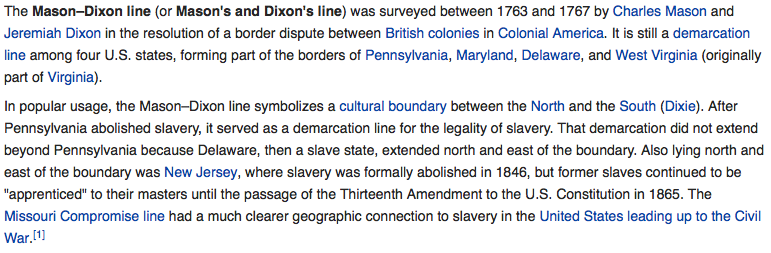## Monday, October 20, 2014

### 47 66 69 | Mason-Dixon Line and Charles MasonA surveyor's chain has 33-links...
Mason = 4+1+1+6+5 = 17
Dixon = 4+9+6+6+5 = 30
Maxon-Dixon = 17+30 = 47
line = 3+9+5+5 = 22
Mason-Dixon Line = 47+22 = 69

also...

Mason = 13+1+19+15+14 = 62
Dixon = 4+9+24+15+14 = 66

Now compare the name Dixon with the name Charles.  Also notice how he finished surveying in 1767... emphasis on 17 and 67...
• Charles = 3+8+1+18+12+5+19 = 66
• Charles = 3+8+1+9+3+5+1 = 30